# GMAT Math : DSQ: Simplifying algebraic expressions

## Example Questions

2 Next →

### Example Question #11 : Dsq: Simplifying Algebraic Expressions

Evaluate the expression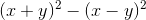Statement 1: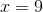Statement 2: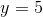BOTH statements TOGETHER are insufficient to answer the question.

EITHER statement ALONE is sufficient to answer the question.

Statement 1 ALONE is sufficient to answer the question, but Statement 2 ALONE is NOT sufficient to answer the question.

Statement 2 ALONE is sufficient to answer the question, but Statement 1 ALONE is NOT sufficient to answer the question.

BOTH statements TOGETHER are sufficient to answer the question, but NEITHER statement ALONE is sufficient to answer the question.

BOTH statements TOGETHER are sufficient to answer the question, but NEITHER statement ALONE is sufficient to answer the question.

Explanation:

We simplify to make sure of whether we need to know one or both ofand: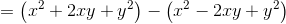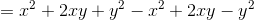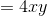Indeed, the values of bothandmust be known to evaluate this expresson.

### Example Question #3012 : Gmat Quantitative Reasoning

Carleton's teacher challenged him to fill in the circle and the square below to form a polynomial with degree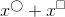Did Carleton succeed?

Statement 1: The sum of the numbers Carleton filled in the two shapes was.

Statement 2: Carleton wrote ain the circle.

EITHER statement ALONE is sufficient to answer the question.

BOTH statements TOGETHER are insufficient to answer the question.

Statement 2 ALONE is sufficient to answer the question, but Statement 1 ALONE is NOT sufficient to answer the question.

Statement 1 ALONE is sufficient to answer the question, but Statement 2 ALONE is NOT sufficient to answer the question.

BOTH statements TOGETHER are sufficient to answer the question, but NEITHER statement ALONE is sufficient to answer the question.

BOTH statements TOGETHER are sufficient to answer the question, but NEITHER statement ALONE is sufficient to answer the question.

Explanation:

The degree of a polynomial is the highest of the degrees of any of its terms; the degree of a term with one variable is the exponent of the variable.

Statement 1 alone provides insufficient information, since, for example, the degreepolynomial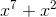and the degree 6 polynomial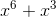both satisfy the statement. Statement 2 alone is also insufficient, since it says nothing about the number in the square.

The two statements together are sufficient; if the number in the circle is, and the numbers add up to, the number in the square is, making the polynomial the degreepolynomial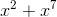.

### Example Question #11 : Dsq: Simplifying Algebraic Expressions

Michelle was challenged by her teacher to create a monomial of degreeby filling in the square and the circle in the figure below.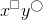Did Michelle succeed?

Statement 1: Michelle wrote the same positive integer in both the circle and the square.

Statement 2: Michelle wrote ain the circle.

BOTH statements TOGETHER are sufficient to answer the question, but NEITHER statement ALONE is sufficient to answer the question.

Statement 2 ALONE is sufficient to answer the question, but Statement 1 ALONE is NOT sufficient to answer the question.

Statement 1 ALONE is sufficient to answer the question, but Statement 2 ALONE is NOT sufficient to answer the question.

BOTH statements TOGETHER are insufficient to answer the question.

EITHER statement ALONE is sufficient to answer the question.

Statement 1 ALONE is sufficient to answer the question, but Statement 2 ALONE is NOT sufficient to answer the question.

Explanation:

The degree of a monomial with more than one variable is the sum of the exponents of the two variables.

If Statement 1 alone is assumed, then, since the same integer is in both shapes, the sum must be twice this integer. The monomial must therefore have even degree—and, specifically, its degree cannot be.

Statement 2 alone proves nothing; if Michelle wrote ain the circle, she could have formed, for example,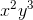, which has degree 5, or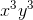, which has degree.

### Example Question #12 : Dsq: Simplifying Algebraic Expressions

Gary was challenged by his teacher to write some whole numbers in the shapes in the diagram below in order to form a simplified expression.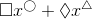Did Gary succeed?

Statement 1: Gary wrote the same whole number in the square and in the diamond.

Statement 2: Gary wrote different whole numbers in the circle and the triangle.

Statement 2 ALONE is sufficient to answer the question, but Statement 1 ALONE is NOT sufficient to answer the question.

Statement 1 ALONE is sufficient to answer the question, but Statement 2 ALONE is NOT sufficient to answer the question.

EITHER statement ALONE is sufficient to answer the question.

BOTH statements TOGETHER are sufficient to answer the question, but NEITHER statement ALONE is sufficient to answer the question.

BOTH statements TOGETHER are insufficient to answer the question.

Statement 2 ALONE is sufficient to answer the question, but Statement 1 ALONE is NOT sufficient to answer the question.

Explanation:

A polynomial in one variable is simplified if and only if no two of its terms are like—that is, if and only if no two terms have the same exponent. Statement 1 alone only addresses the coefficients, which are not relevant to whether the expression can be simplified further. By Statement 2 alone, Gary wrote different exponents, so he succeeded in forming a simplified polynomial.

### Example Question #11 : Simplifying Algebraic Expressions

Evaluate the expression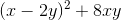.

Statement 1: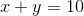Statement 2: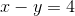Statement 2 ALONE is sufficient to answer the question, but Statement 1 ALONE is NOT sufficient to answer the question.

Statement 1 ALONE is sufficient to answer the question, but Statement 2 ALONE is NOT sufficient to answer the question.

BOTH statements TOGETHER are sufficient to answer the question, but NEITHER statement ALONE is sufficient to answer the question.

BOTH statements TOGETHER are insufficient to answer the question.

EITHER statement ALONE is sufficient to answer the question.

BOTH statements TOGETHER are sufficient to answer the question, but NEITHER statement ALONE is sufficient to answer the question.

Explanation:

Simplify the expression: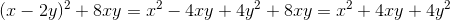This is equal to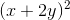Knowing the sum or the difference ofandis not enough to determine the value of this expression. But knowing both allows you to solve a system of equations:____________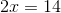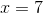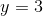The expression can now be evaluated by substitution.

The answer is that both statements together are sufficient to answer the question, but neither statement alone is sufficient to answer the question.

2 Next →

### All GMAT Math Resources